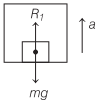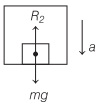# What is Weight in Physics | Definition, Example – Laws of Motion

## What is Weight in Physics | Definition, Example – Laws of Motion

We are giving a detailed and clear sheet on all Physics Notes that are very useful to understand the Basic Physics Concepts.

Weight Definition Physics (w):
It is a field force. It is the force with which a body is pulled towards the centre of the earth due to gravity. It has the magnitude mg, where m is the mass of the body and g is the acceleration due to gravity.

w = mg

Normal Reaction
It is a contact force. It is the force between two surfaces in contact, which is always perpendicular to the surfaces in contact.

Tension

• Tension force always pulls a body.
• Tension is a reactive force. It is not an active force.
• Tension across a massless pulley or frictionless pulley remains constant.
• Rope becomes slack when tension force becomes zero.

Apparent Weight in Lift
(i) When a lift is at rest or moving with a constant speed, thenThe weighing machine will read the actual weight.

(ii) When a lift is accelerating upwards, then apparent weight

R1 = m(g + a)The weighing machine will read the apparent weight, which is more than the actual weight.

(iii) When a lift is accelerating downwards, then apparent weight

R2 = m(g-a)The weighing machine will read the apparent weight, which is less than the actual weight.

(iv) When lift is falling freely under gravity, then

R2 = m(g-g) = 0

The apparent weight of the body becomes zero.

(v) If lift is accelerating downward with an acceleration greater than g, then body will be lifted from floor to the ceiling of the lift.

Laws of Motion:
There are various laws in Physics that define the motion of the object. When an object is in motion whether it is linear or circular there is some force which is always imposed on it.